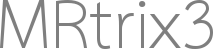# Interpretation of FBA non-group-comparison absolute effect size

Hi @rsmith,

Thanks for the useful wiki entry. I was wondering how the abs_effect_size and beta coefficient files are determined for when you have a more complicated situation that is not simply patient vs control.

For example,

I have a design matrix that looks like this:
1 0.0644860566627119 -1
1 2.8305030911727336 -1
1 -1.4999006267896773 -1
1 -0.887749315873525 -1
1 1.5098433185480715 -1
1 1.0167214291989488 -1
1 0.1381709366803969 -1
1 -1.4942325590960093 -1
1 1.5891962662594246 -1

The first column is the global intercept, the second is the age (de-meaned and divided by the standard deviation), and the third column is the sex (1 for M, -1 for F)

The line in my contrast matrix I am most interested in is

[0 -1 0], so essentially testing for a negative corr. between FD/FDC/log FC and age, if I’m getting that right.

In this case, how can one interpret the absolute_effect_size .mif files?

Thanks so much!

1 Like

Hi Ana,

I’ll give this question its own topic instead, since it’s slightly different to the “tricks” that are currently present in that Wiki page; but it’s a great question, involving data manipulations that I’ve had to do myself for one of my own experiments, and something that should ideally appear if I ever find the time to write a more general GLM wiki page.

What the beta coefficients provided by the GLM encode is the gradient between the predicted values of the exploratory variable (i.e. the imaging metric data you provide as input) and the explanatory variable (the column of values in the GLM).

Imagine that you have some nuisance regressor X, and that the value of the beta coefficient corresponding to the X column is 2.0. And let’s say you’re testing FDC for simplicity. What that means is that the linear model predicts that if, for some hypothetical subject, the value in the X column were to increase by 1.0, the value of FDC would increase by 2.0.

In your case, X is actually: `((age - mean_age) / std_age)`.
So, if the second beta coefficient is 2.0, that means that if `((age - mean_age) / std_age)` were to increase by 1.0, FDC would increase by 2.0.
Therefore, if you multiply the value of that beta coefficient by `(std_age)`, what that gives you is the model’s prediction of the rate of change of FDC with respect to age!
(The subtraction disappears; we’re only interested in rate of change, and the age offset gets gobbled up as part of the global intercept term; but you can use the same logic to derive the complete estimated linear relationship with respect to age)

Now I’ve explained all of this in terms of beta coefficients. But the absolute effect is simply the inner product between the beta coefficients and the contrast matrix. Since your contrast is `[0 -1 0]`, what file `abs_effect_size.mif` is actually providing you with is: `-((age - mean_age) / std_age)` (note the negative!). So you would want to multiply by `(-std_age)` to get the rate of change of FDC with respect to age, as opposed to the negative of such.

This isn’t actually any different to the interpretation of these values in a group difference context!

For instance, let’s say that you’re also interested in the magnitude of the sex effect in your cohort. Internally, the GLM doesn’t care that the values in that third column are all +1 or -1; it still treats it as a continuous distribution. What the third beta coefficient provides you with is the rate of change of FDC with respect to Y, where Y is a dummy variable “encoding” sex. But a unitary change in Y is only half of the difference between female and male; i.e. the “distance” between female and male in the dimension of variable Y is 2.0. Therefore, if you actually wanted to know what the model predicts to be the difference in FDC between an exemplar male and an exemplar female (additionally regressing for all other variables), you would need to double the corresponding beta coefficient!

(I’ve actually wondered about advocating the use of `-0.5` and `0.5` for categorical dummy variables for this reason…)

Cheers
Rob